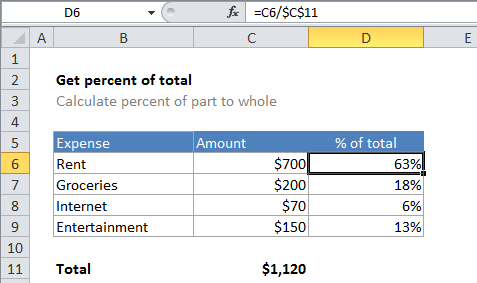# How to calculate percentage of total in Excel

To calculate percent of a total (i.e. calculate a percent distribution), you can use a formula that simply divides an amount by the total.

## Formula

`=amount/total`## Explanation

In the example shown, the formula in D6 is:

`=C6/\$C\$11`

Note: the result is formatted with Percentage number format to show 25%, 10%, etc.

## How the formula works

In the example, D6 contains the formula below, copied down:

`=C6/\$C\$11`

In cell D6, the result of the calculation is .625. The Percentage number format is applied to cell D6, with 2 decimal places, so Excel displays .625 as 63%.

Also See:   Superscript and Subscript in Excel

The reference to C11 is absolute (i.e. \$C\$11) so that it won’t change when the formula is copied down column D. This means that each value in column C will be divided by the same total in C11.

### Percentage vs number

When you want to display a percentage in Excel, use the Percentage number format, which will automatically display a decimal value as a percentage. If you want a simple number instead without a percent sign (i.e. 75, 85, 100, etc.) you can multiply by 100:

`=(C6/\$C\$11)*100`